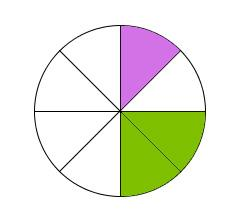# One-seventh 3214

Determine one-third of the number, one-fifth of which is one-seventh.

x =  0.2381

### Step-by-step explanation:

n/5 = 1/7
x = n/3

7n = 5
n-3x = 0

Row 2 - 1/7 · Row 1 → Row 2
7n = 5
-3x = -0.71

x = -0.71428571/-3 = 0.23809524
n = 5/7 = 0.71428571

n = 5/7 ≈ 0.714286
x = 5/21 ≈ 0.238095

Our linear equations calculator calculates it.Did you find an error or inaccuracy? Feel free to write us. Thank you!

Tips for related online calculators
Need help calculating sum, simplifying, or multiplying fractions? Try our fraction calculator.
Do you have a linear equation or system of equations and looking for its solution? Or do you have a quadratic equation?